GFG App
Open AppBrowser
Continue

# Decision Making in C / C++ (if , if..else, Nested if, if-else-if )

The conditional statements (also known as decision control structures) such as if, if else, switch, etc. are used for decision-making purposes in C/C++ programs.

They are also known as Decision-Making Statements and are used to evaluate one or more conditions and make the decision whether to execute a set of statements or not. These decision-making statements in programming languages decide the direction of the flow of program execution.

## Need of Conditional Statements

There come situations in real life when we need to make some decisions and based on these decisions, we decide what should we do next. Similar situations arise in programming also where we need to make some decisions and based on these decisions we will execute the next block of code. For example, in C if x occurs then execute y else execute z. There can also be multiple conditions like in C if x occurs then execute p, else if condition y occurs execute q, else execute r. This condition of C else-if is one of the many ways of importing multiple conditions.

## Types of Conditional Statements in C/C++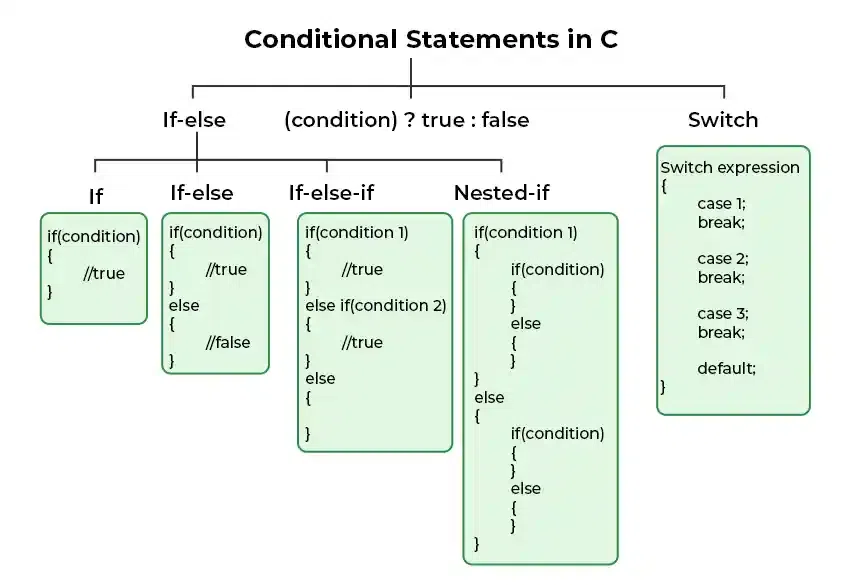Following are the decision-making statements available in C or C++:

1. if Statement
2. if-else Statement
3. Nested if Statement
5. switch Statement
6. Conditional Operator
7. Jump Statements:
• break
• continue
• goto
• return

Let’s discuss each of them one by one.

## 1. if in C/C++

The if statement is the most simple decision-making statement. It is used to decide whether a certain statement or block of statements will be executed or not i.e if a certain condition is true then a block of statements is executed otherwise not.

### Syntax of if Statement

```if(condition)
{
// Statements to execute if
// condition is true
}```

Here, the condition after evaluation will be either true or false. C if statement accepts boolean values – if the value is true then it will execute the block of statements below it otherwise not. If we do not provide the curly braces ‘{‘ and ‘}’ after if(condition) then by default if statement will consider the first immediately below statement to be inside its block.

### Flowchart of if Statement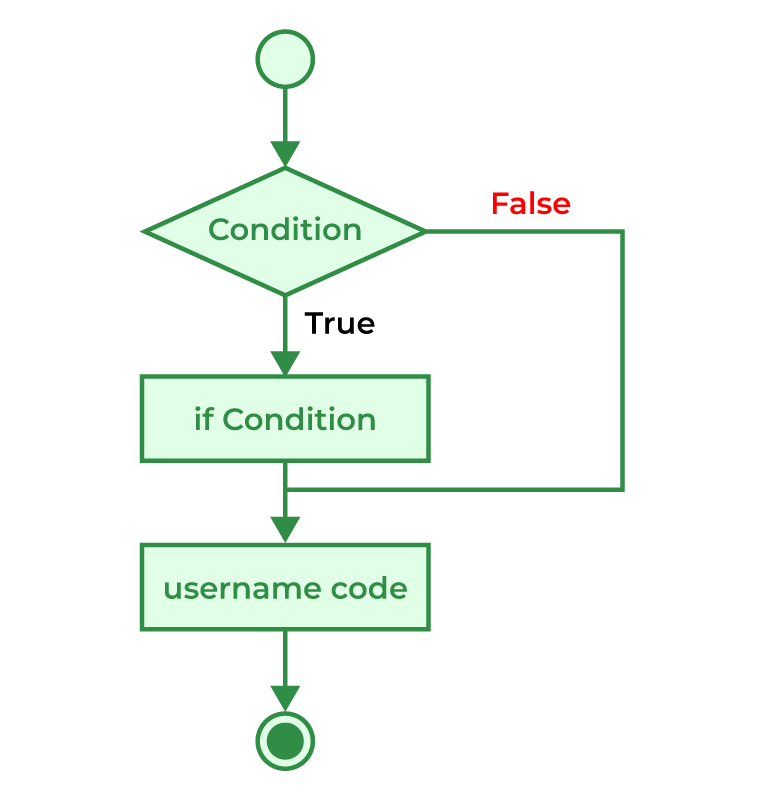Flow Diagram of if Statement

## C

 `// C program to illustrate If statement` `#include `   `int` `main()` `{` `    ``int` `i = 10;`   `    ``if` `(i > 15) {` `        ``printf``(``"10 is greater than 15"``);` `    ``}`   `    ``printf``(``"I am Not in if"``);` `}`

## C++

 `// C++ program to illustrate If statement` `#include ` `using` `namespace` `std;`   `int` `main()` `{` `    ``int` `i = 10;`   `    ``if` `(i > 15) {` `        ``cout << ``"10 is greater than 15"``;` `    ``}`   `    ``cout << ``"I am Not in if"``;` `}`

Output

`I am Not in if`

As the condition present in the if statement is false. So, the block below the if statement is not executed.

## 2. if-else in C/C++

The if statement alone tells us that if a condition is true it will execute a block of statements and if the condition is false it won’t. But what if we want to do something else when the condition is false? Here comes the C else statement. We can use the else statement with the if statement to execute a block of code when the condition is false. The if-else statement consists of two blocks, one for false expression and one for true expression.

### Syntax of if else in C/C++

```if (condition)
{
// Executes this block if
// condition is true
}
else
{
// Executes this block if
// condition is false
}```

### Flowchart of if-else Statement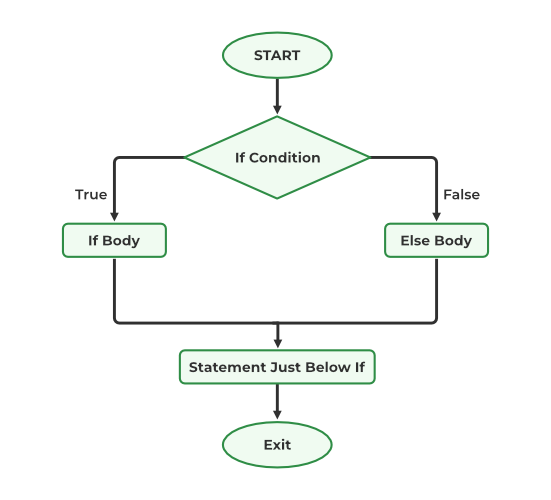Flow Diagram of if else

## C

 `// C program to illustrate If statement` `#include `   `int` `main()` `{` `    ``int` `i = 20;`   `    ``if` `(i < 15) {`   `        ``printf``(``"i is smaller than 15"``);` `    ``}` `    ``else` `{`   `        ``printf``(``"i is greater than 15"``);` `    ``}` `    ``return` `0;` `}`

## C++

 `// C++ program to illustrate if-else statement` `#include ` `using` `namespace` `std;`   `int` `main()` `{` `    ``int` `i = 20;`   `    ``if` `(i < 15)` `        ``cout << ``"i is smaller than 15"``;` `    ``else` `        ``cout << ``"i is greater than 15"``;`   `    ``return` `0;` `}`

Output

`i is greater than 15`

The block of code following the else statement is executed as the condition present in the if statement is false.

## 3. Nested if-else in C/C++

A nested if in C is an if statement that is the target of another if statement. Nested if statements mean an if statement inside another if statement. Yes, both C and C++ allow us to nested if statements within if statements, i.e, we can place an if statement inside another if statement.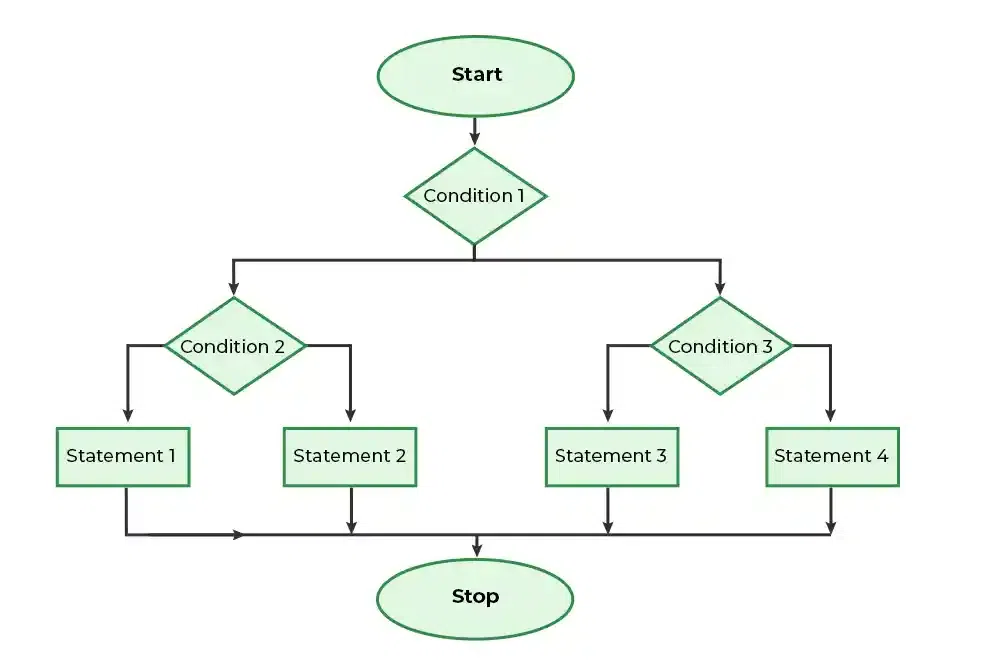Flow Diagram of Nested if-else

### Syntax of Nested if-else

```if (condition1)
{
// Executes when condition1 is true
if (condition2)
{
// Executes when condition2 is true
}
else
{
// Executes when condition2 is false
}```

//IMAGE

## C

 `// C program to illustrate nested-if statement` `#include `   `int` `main()` `{` `    ``int` `i = 10;`   `    ``if` `(i == 10) {` `        ``// First if statement` `        ``if` `(i < 15)` `            ``printf``(``"i is smaller than 15\n"``);`   `        ``// Nested - if statement` `        ``// Will only be executed if statement above` `        ``// is true` `        ``if` `(i < 12)` `            ``printf``(``"i is smaller than 12 too\n"``);` `        ``else` `            ``printf``(``"i is greater than 15"``);` `    ``}`   `    ``return` `0;` `}`

## C++

 `// C++ program to illustrate nested-if statement` `#include ` `using` `namespace` `std;`   `int` `main()` `{` `    ``int` `i = 10;`   `    ``if` `(i == 10) {` `        ``// First if statement` `        ``if` `(i < 15)` `            ``cout << ``"i is smaller than 15\n"``;`   `        ``// Nested - if statement` `        ``// Will only be executed if ` `        ``// statement above is true` `        ``if` `(i < 12)` `            ``cout << ``"i is smaller than 12 too\n"``;` `        ``else` `            ``cout << ``"i is greater than 15"``;` `    ``}`   `    ``return` `0;` `}`

Output

```i is smaller than 15
i is smaller than 12 too```

## 4. if-else-if Ladder in C/C++

The if else if statements are used when the user has to decide among multiple options. The C if statements are executed from the top down. As soon as one of the conditions controlling the if is true, the statement associated with that if is executed, and the rest of the C else-if ladder is bypassed. If none of the conditions is true, then the final else statement will be executed. if-else-if ladder is similar to the switch statement.

```if (condition)
statement;
else if (condition)
statement;
.
.

else
statement;```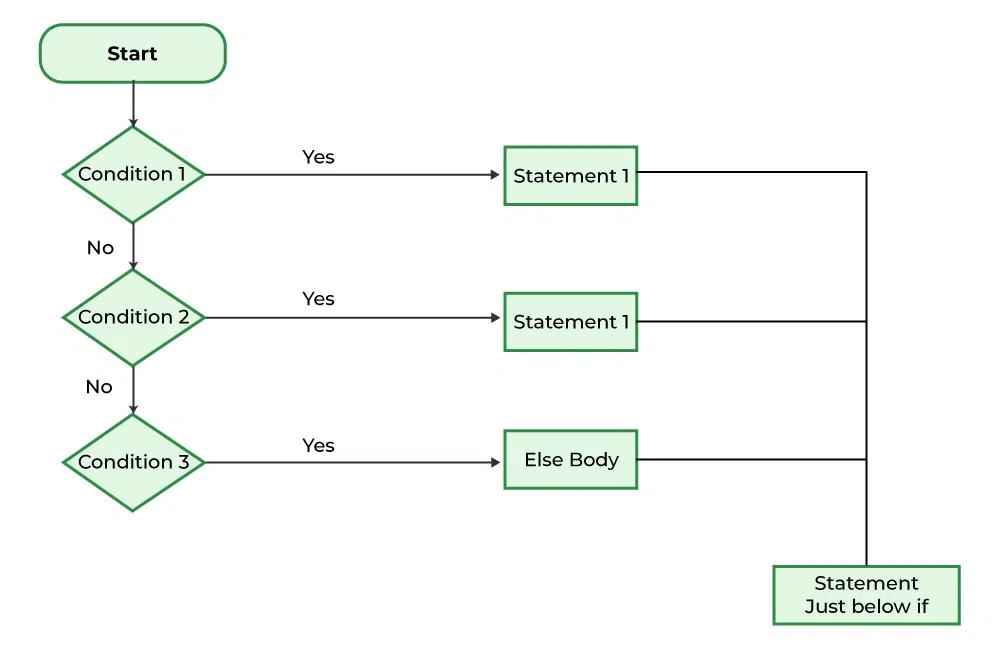Flow Diagram of if-else-if

## C

 `// C program to illustrate nested-if statement` `#include `   `int` `main()` `{` `    ``int` `i = 20;`   `    ``if` `(i == 10)` `        ``printf``(``"i is 10"``);` `    ``else` `if` `(i == 15)` `        ``printf``(``"i is 15"``);` `    ``else` `if` `(i == 20)` `        ``printf``(``"i is 20"``);` `    ``else` `        ``printf``(``"i is not present"``);` `}`

## C++

 `// C++ program to illustrate if-else-if ladder` `#include ` `using` `namespace` `std;`   `int` `main()` `{` `    ``int` `i = 20;`   `    ``if` `(i == 10)` `        ``cout << ``"i is 10"``;` `    ``else` `if` `(i == 15)` `        ``cout << ``"i is 15"``;` `    ``else` `if` `(i == 20)` `        ``cout << ``"i is 20"``;` `    ``else` `        ``cout << ``"i is not present"``;` `}`

Output

`i is 20`

## 5. switch Statement in C/C++

The switch case statement is an alternative to the if else if ladder that can be used to execute the conditional code based on the value of the variable specified in the switch statement. The switch block consists of cases to be executed based on the value of the switch variable.

### Syntax of switch

```switch (expression) {
case value1:
statements;
case value2:
statements;
....
....
....
default:
statements;
}```

Note: The switch expression should evaluate to either integer or character. It cannot evaluate any other data type.

### Flowchart of switch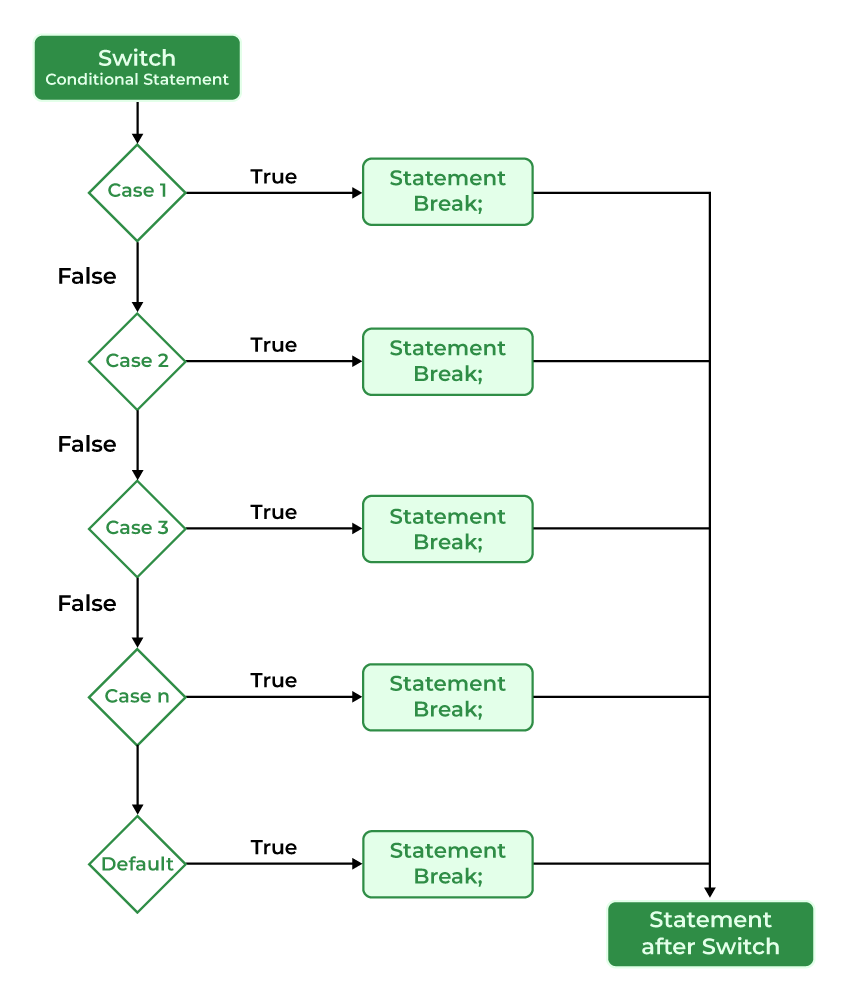Flow Diagram of switch in C/C++

## C

 `// C Program to illustrate the use of switch statement` `#include `   `int` `main()` `{` `    ``// variable to be used in switch statement` `    ``int` `var = 2;`   `    ``// declaring switch cases` `    ``switch` `(var) {` `    ``case` `1:` `        ``printf``(``"Case 1 is executed"``);` `        ``break``;` `    ``case` `2:` `        ``printf``(``"Case 2 is executed"``);` `        ``break``;` `    ``default``:` `        ``printf``(``"Default Case is executed"``);` `        ``break``;` `    ``}`   `    ``return` `0;` `}`

## C++

 `// C Program to illustrate the use of switch statement` `#include ` `using` `namespace` `std;`   `// driver code` `int` `main()` `{` `    ``// variable to be used in switch statement` `    ``int` `var = 2;`   `    ``// declaring switch cases` `    ``switch` `(var) {` `    ``case` `1:` `        ``cout << ``"Case 1 is executed"``;` `        ``break``;` `    ``case` `2:` `        ``cout << ``"Case 2 is executed"``;` `        ``break``;` `    ``default``:` `        ``cout << ``"Default Case is executed"``;` `        ``break``;` `    ``}`   `    ``return` `0;` `}`

Output

`Case 2 is executed`

## 6. Conditional Operator in C/C++

The conditional operator is used to add conditional code in our program. It is similar to the if-else statement. It is also known as the ternary operator as it works on three operands.

### Syntax of Conditional Operator

`(condition) ? [true_statements] : [flase_statements];`

### Flowchart of Conditional Operator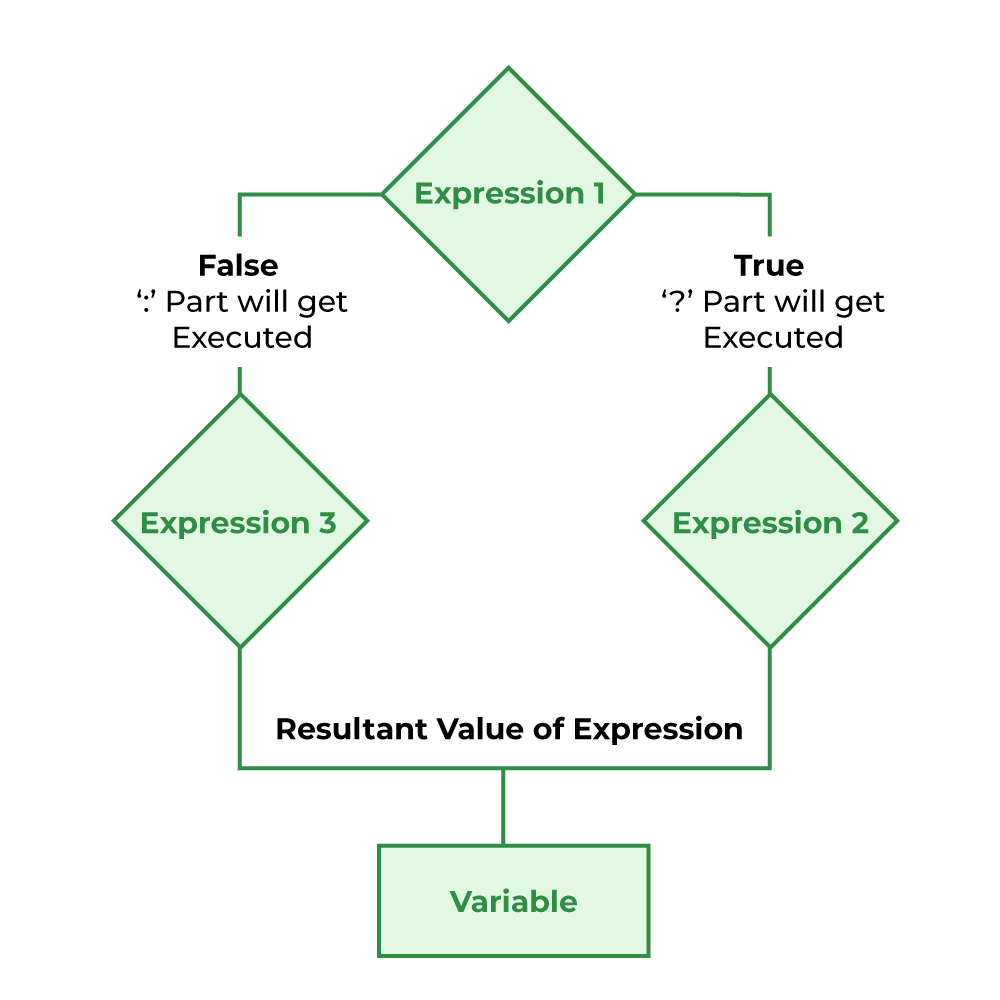Flow Diagram of Conditional Operator

## C

 `// C Program to illustrate the use of conditional operator` `#include `   `// driver code` `int` `main()` `{`   `    ``int` `var;` `    ``int` `flag = 0;`   `    ``// using conditional operator to assign the value to var` `    ``// according to the value of flag` `    ``var = flag == 0 ? 25 : -25;` `    ``printf``(``"Value of var when flag is 0: %d\n"``, var);`   `    ``// changing the value of flag` `    ``flag = 1;` `    ``// again assigning the value to var using same statement` `    ``var = flag == 0 ? 25 : -25;` `    ``printf``(``"Value of var when flag is NOT 0: %d"``, var);`   `    ``return` `0;` `}`

## C++

 `// C++ Program to illustrate the use of conditional operator` `#include ` `using` `namespace` `std;`   `// driver code` `int` `main()` `{`   `    ``int` `var;` `    ``int` `flag = 0;`   `    ``// using conditional operator to assign the value to var` `    ``// according to the value of flag` `    ``var = flag == 0 ? 25 : -25;` `    ``cout << ``"Value of var when flag is 0: "` `<< var << endl;`   `    ``// changing the value of flag` `    ``flag = 1;` `    ``// again assigning the value to var using same statement` `    ``var = flag == 0 ? 25 : -25;` `    ``cout << ``"Value of var when flag is NOT 0: "` `<< var;`   `    ``return` `0;` `}`

Output

```Value of var when flag is 0: 25
Value of var when flag is NOT 0: -25```

## 7. Jump Statements in C/C++

These statements are used in C or C++ for the unconditional flow of control throughout the functions in a program. They support four types of jump statements:

### A) break

This loop control statement is used to terminate the loop. As soon as the break statement is encountered from within a loop, the loop iterations stop there, and control returns from the loop immediately to the first statement after the loop.

#### Syntax of break

`break;`

Basically, break statements are used in situations when we are not sure about the actual number of iterations for the loop or we want to terminate the loop based on some condition.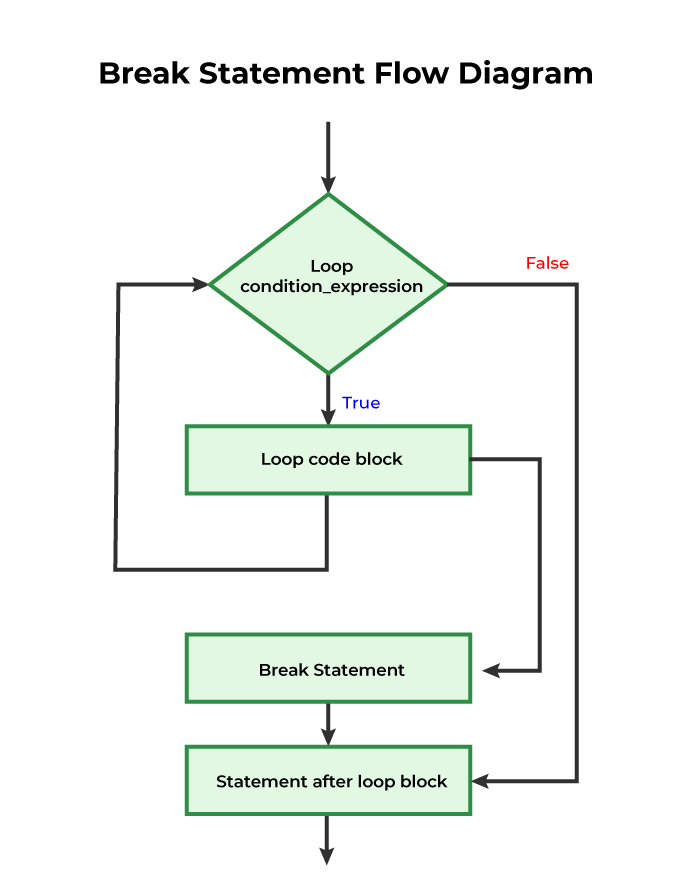## C

 `// C program to illustrate` `// to show usage of break` `// statement` `#include `   `void` `findElement(``int` `arr[], ``int` `size, ``int` `key)` `{` `    ``// loop to traverse array and search for key` `    ``for` `(``int` `i = 0; i < size; i++) {` `        ``if` `(arr[i] == key) {` `            ``printf``(``"Element found at position: %d"``,` `                   ``(i + 1));` `            ``break``;` `        ``}` `    ``}` `}`   `int` `main()` `{` `    ``int` `arr[] = { 1, 2, 3, 4, 5, 6 };`   `    ``// no of elements` `    ``int` `n = 6;`   `    ``// key to be searched` `    ``int` `key = 3;`   `    ``// Calling function to find the key` `    ``findElement(arr, n, key);`   `    ``return` `0;` `}`

## C++

 `// C++ program to illustrate` `// to show usage of break` `// statement` `#include ` `using` `namespace` `std;`   `void` `findElement(``int` `arr[], ``int` `size, ``int` `key)` `{` `    ``// loop to traverse array and search for key` `    ``for` `(``int` `i = 0; i < size; i++) {` `        ``if` `(arr[i] == key) {` `            ``cout << ``"Element found at position: "` `                 ``<< (i + 1);` `            ``break``;` `        ``}` `    ``}` `}`   `// Driver program to test above function` `int` `main()` `{` `    ``int` `arr[] = { 1, 2, 3, 4, 5, 6 };` `    ``int` `n = 6; ``// no of elements` `    ``int` `key = 3; ``// key to be searched`   `    ``// Calling function to find the key` `    ``findElement(arr, n, key);`   `    ``return` `0;` `}`

Output

`Element found at position: 3`

### B) continue

This loop control statement is just like the break statement. The continue statement is opposite to that of the break statement, instead of terminating the loop, it forces to execute the next iteration of the loop.
As the name suggests the continue statement forces the loop to continue or execute the next iteration. When the continue statement is executed in the loop, the code inside the loop following the continue statement will be skipped and the next iteration of the loop will begin.

#### Syntax of continue

`continue;`

#### Flowchart of Continue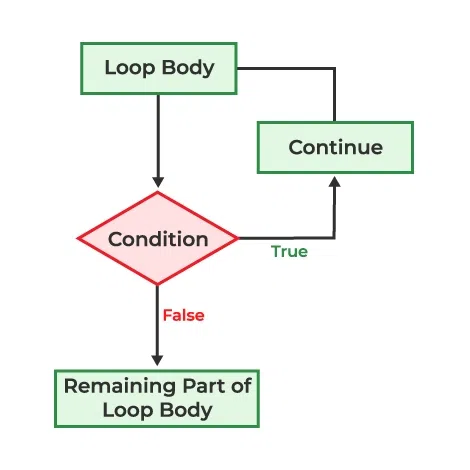Flow Diagram of C continue Statement

## C

 `// C program to explain the use` `// of continue statement` `#include `   `int` `main()` `{` `    ``// loop from 1 to 10` `    ``for` `(``int` `i = 1; i <= 10; i++) {`   `        ``// If i is equals to 6,` `        ``// continue to next iteration` `        ``// without printing` `        ``if` `(i == 6)` `            ``continue``;`   `        ``else` `            ``// otherwise print the value of i` `            ``printf``(``"%d "``, i);` `    ``}`   `    ``return` `0;` `}`

## C++

 `// C++ program to explain the use` `// of continue statement`   `#include ` `using` `namespace` `std;`   `int` `main()` `{` `    ``// loop from 1 to 10` `    ``for` `(``int` `i = 1; i <= 10; i++) {`   `        ``// If i is equals to 6,` `        ``// continue to next iteration` `        ``// without printing` `        ``if` `(i == 6)` `            ``continue``;`   `        ``else` `            ``// otherwise print the value of i` `            ``cout << i << ``" "``;` `    ``}`   `    ``return` `0;` `}`

Output

`1 2 3 4 5 7 8 9 10 `

If you create a variable in if-else in C/C++, it will be local to that if/else block only. You can use global variables inside the if/else block. If the name of the variable you created in if/else is as same as any global variable then priority will be given to the `local variable`.

## C

 `#include `   `int` `main()` `{`   `    ``int` `gfg = 0; ``// local variable for main` `    ``printf``(``"Before if-else block %d\n"``, gfg);` `    ``if` `(1) {` `        ``int` `gfg = 100; ``// new local variable of if block` `        ``printf``(``"if block %d\n"``, gfg);` `    ``}` `    ``printf``(``"After if block %d"``, gfg);` `    ``return` `0;` `}`

## C++

 `#include ` `using` `namespace` `std;`   `int` `main()` `{` `    ``int` `gfg = 0; ``// local variable for main` `    ``cout << ``"Before if-else block "` `<< gfg << endl;` `    ``if` `(1) {` `        ``int` `gfg = 100; ``// new local variable of if block` `        ``cout << ``"if block "` `<< gfg << endl;` `    ``}` `    ``cout << ``"After if block "` `<< gfg << endl;` `    ``return` `0;` `}` `/*` `    ``Before if-else block 0` `    ``if block 100` `    ``After if block 0` `*/`

Output

```Before if-else block 0
if block 100
After if block 0```

### C) goto

The goto statement in C/C++ also referred to as the unconditional jump statement can be used to jump from one point to another within a function.

#### Syntax of goto

```Syntax1      |   Syntax2
----------------------------
goto label;  |    label:
.            |    .
.            |    .
.            |    .
label:       |    goto label;```

In the above syntax, the first line tells the compiler to go to or jump to the statement marked as a label. Here, a label is a user-defined identifier that indicates the target statement. The statement immediately followed after ‘label:’ is the destination statement. The ‘label:’ can also appear before the ‘goto label;’ statement in the above syntax.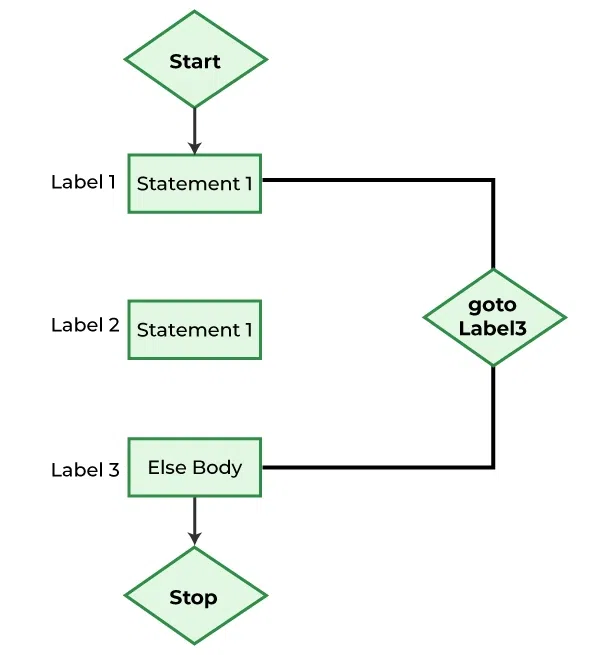Flowchart of goto Statement

## C

 `// C program to print numbers` `// from 1 to 10 using goto ` `// statement` `#include `   `// function to print numbers from 1 to 10` `void` `printNumbers()` `{` `    ``int` `n = 1;` `label:` `    ``printf``(``"%d "``, n);` `    ``n++;` `    ``if` `(n <= 10)` `        ``goto` `label;` `}`   `// Driver program to test above function` `int` `main()` `{` `    ``printNumbers();` `    ``return` `0;` `}`

## C++

 `// C++ program to print numbers` `// from 1 to 10 using goto` `// statement` `#include ` `using` `namespace` `std;`   `// function to print numbers from 1 to 10` `void` `printNumbers()` `{` `    ``int` `n = 1;` `label:` `    ``cout << n << ``" "``;` `    ``n++;` `    ``if` `(n <= 10)` `        ``goto` `label;` `}`   `// Driver program to test above function` `int` `main()` `{` `    ``printNumbers();` `    ``return` `0;` `}`

Output

`1 2 3 4 5 6 7 8 9 10 `

### D) return

The return in C or C++ returns the flow of the execution to the function from where it is called. This statement does not mandatorily need any conditional statements. As soon as the statement is executed, the flow of the program stops immediately and returns the control from where it was called. The return statement may or may not return anything for a void function, but for a non-void function, a return value must be returned.

#### Flowchart of return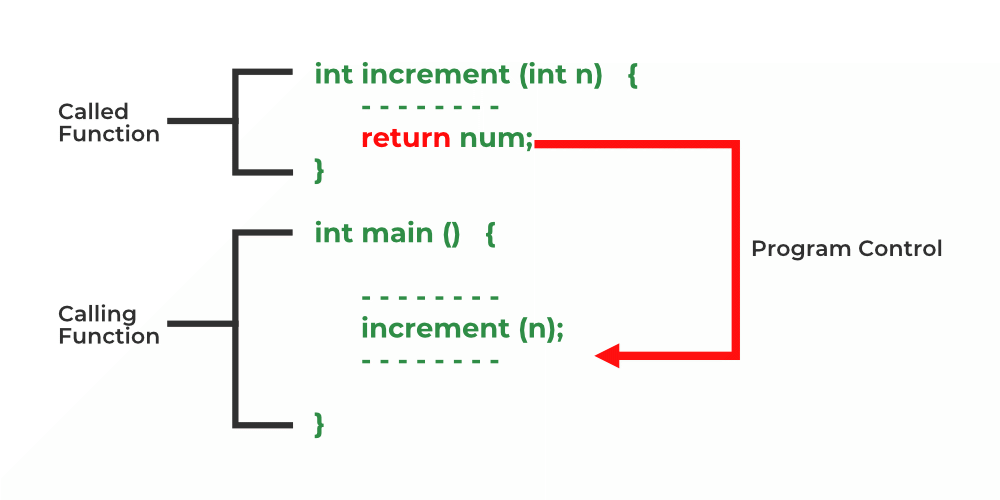Flow Diagram of return

#### Syntax of return

`return [expression];`

## C

 `// C code to illustrate return` `// statement` `#include `   `// non-void return type` `// function to calculate sum` `int` `SUM(``int` `a, ``int` `b)` `{` `    ``int` `s1 = a + b;` `    ``return` `s1;` `}`   `// returns void` `// function to print` `void` `Print(``int` `s2)` `{` `    ``printf``(``"The sum is %d"``, s2);` `    ``return``;` `}`   `int` `main()` `{` `    ``int` `num1 = 10;` `    ``int` `num2 = 10;` `    ``int` `sum_of = SUM(num1, num2);` `    ``Print(sum_of);` `    ``return` `0;` `}`

## C++

 `// C++ code to illustrate return` `// statement` `#include ` `using` `namespace` `std;`   `// non-void return type` `// function to calculate sum` `int` `SUM(``int` `a, ``int` `b)` `{` `    ``int` `s1 = a + b;` `    ``return` `s1;` `}`   `// returns void` `// function to print` `void` `Print(``int` `s2)` `{` `    ``cout << ``"The sum is "` `<< s2;` `    ``return``;` `}`   `int` `main()` `{` `    ``int` `num1 = 10;` `    ``int` `num2 = 10;` `    ``int` `sum_of = SUM(num1, num2);` `    ``Print(sum_of);` `    ``return` `0;` `}`

Output

`The sum is 20`

My Personal Notes arrow_drop_up